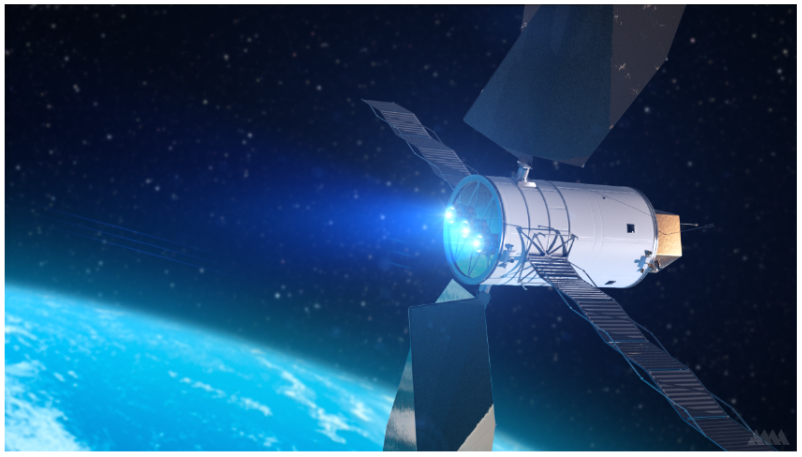# Applications of Calculus: Rocket PropulsionWhat speed can a rocket reach if it has a particular amount of fuel? This is a difficult question because when a rocket burns some amount of fuel, it gets lighter, so burning the same amount of fuel again will speed the rocket up even more. But calculus can help us answer it.

Let’s think of a rocket burning a small bit of fuel and speeding up by a small bit. We have the following variables:

• $\Delta m =$ mass of small bit of fuel to be burnt
• $m =$ mass of fueled rocket after burning the bit of fuel
• $v =$ velocity of fueled rocket before burning the bit of fuel
• $\Delta v =$ small increase in velocity due to burning the small bit of fuel
• $u =$ velocity of fuel exhaust, from the rocket's frame of reference

The law of conservation of momentum tells us that the momentum (mass times velocity) of the rocket and fuel should stay the same even after a bit of fuel is burnt.

The momentum before the fuel is burnt is obtained by multiplying the mass of the fueled rocket (including the small bit of fuel that hasn’t been burnt yet) with the velocity of the fueled rocket before burning the fuel. So, it is

\begin{align*} (m + \Delta m)v. \end{align*}

The momentum of the rocket after the fuel is burnt is obtained by multiplying the mass of the fueled rocket (excluding the small bit of fuel that was burnt) with the slightly increased velocity of the fueled rocket after burning the fuel. So, it is

\begin{align*} m(v + \Delta v). \end{align*}

However, the burnt bit of fuel also shoots away from the rocket as exhaust, giving additional momentum

\begin{align*} \Delta m(v - u). \end{align*}

Consequently, the total momentum after burning fuel is

\begin{align*} m(v + \Delta v) + \Delta m(v - u). \end{align*}

Setting momentum before fuel-burning equal to momentum after fuel-burning, we have

\begin{align*} (m + \Delta m)v &= m(v + \Delta v) + \Delta m (v-u) \\[5pt] mv + \Delta m v &= mv + m \Delta v + \Delta m v = \Delta m u \\[5pt] m \Delta v &= u \Delta m \end{align*}

In the limit as the amount of burnt fuel gets infinitely small, we can replace the deltas with differentials. Since ejecting a positive $\Delta m$ results in a negative change in mass for the rocket, we use $\Delta m = - dm.$

\begin{align*} m dv = -u dm \end{align*}

Finally, we separate variables and solve the differential equation. (Keep in mind that $u$ is constant.)

\begin{align*} \int dv &= -u \int \frac{dm}{m} \\[5pt] v_{final}-v_{initial} &= -u \left[ \ln(m) \right]^{m_{final}}_{m_{initial}} \\[5pt] v_{final} &= v_{initial} -u \left[ \ln(m_{final}) - \ln(m_{initial}) \right] \\[5pt] v_{final} &= v_{initial} + u \left[ \ln(m_{initial}) - \ln(m_{final}) \right] \\[5pt] v_{final} &= v_{initial} +u \ln \left( \frac{m_{initial}}{m_{final}} \right) \end{align*}

This is called the ideal rocket equation, which can be applied to orbital maneuvers in order to determine how much fuel is needed to change to a particular new orbit, or to find the new orbit as the result of burning some amount of fuel.

Tags: